Courses

# Test: MOSFETs Circuits At DC

## 10 Questions MCQ Test Electronic Devices | Test: MOSFETs Circuits At DC

Description
This mock test of Test: MOSFETs Circuits At DC for Electrical Engineering (EE) helps you for every Electrical Engineering (EE) entrance exam. This contains 10 Multiple Choice Questions for Electrical Engineering (EE) Test: MOSFETs Circuits At DC (mcq) to study with solutions a complete question bank. The solved questions answers in this Test: MOSFETs Circuits At DC quiz give you a good mix of easy questions and tough questions. Electrical Engineering (EE) students definitely take this Test: MOSFETs Circuits At DC exercise for a better result in the exam. You can find other Test: MOSFETs Circuits At DC extra questions, long questions & short questions for Electrical Engineering (EE) on EduRev as well by searching above.
QUESTION: 1

### The transistor in the circuit shown below has kn = 0.4 mA/V2, Vt = 0.5 V and λ = 0. Operation at the edge of saturation is obtained when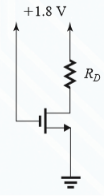Solution:

Use the standard formula for edge saturation.

QUESTION: 2

### The PMOS transistor in the circuit shown has Vt = −0.7 V, μpCox = 60 μA/V2, L = 0.8 μm, and λ = 0. Find the value of R in order to establish a drain current of 0.115 mA and a voltage VD of 3.5 V.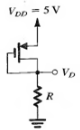Solution: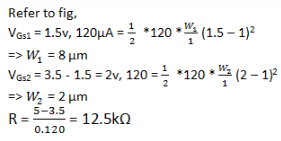QUESTION: 3

### The NMOS transistors in the circuit shown have Vt = 1 V, μnCOX = 120 μA/V2, λ = 0, and L1= L2 = L3 = 1μm. Then which of the following is not the value of the width of these MOSFETs shown

Solution: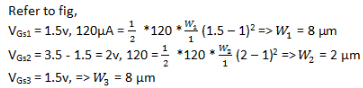QUESTION: 4

The MOSFET shown has Vt = 1V, kn = 100µA/V2 and λ = 0. Find the required values of W/L and of R so that when vI = VDD = +5 V, rDS = 50 Ω, and VO = 50 mV.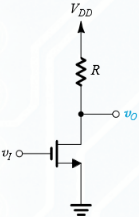Solution:
QUESTION: 5

(Q.5-Q.7) For each of the circuits shown find the labeled voltages. For all transistors, kn(W/L) = 1 mA/V2, Vt = 2V, and λ = 0
5. Find V3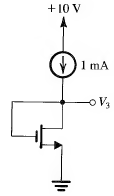Solution:

V3 = 10- 4 * 2 + 1.4 = 3.4v.

QUESTION: 6

Find V4 and V5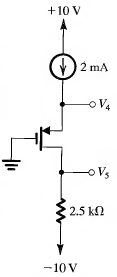Solution:
QUESTION: 7

Find V1 and V2

Solution:

ID = 1 = 12 * 1 * (VGS – 2)2 => VGS = 3.41v.
V3 = 3.41v.

QUESTION: 8

(Q.8 & Q.9) For each of the circuits shown find the labeled node voltages. The NMOS transistors have Vt = 1 V and kn( W/L ) = 2 mA/V2 and λ = 0
Find V1 and V2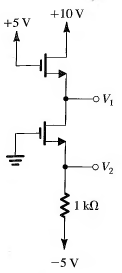Solution:
QUESTION: 9

Find V3 and V4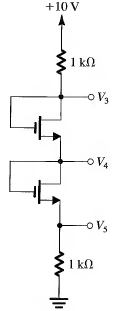Solution:
QUESTION: 10

For the PMOS transistor in the circuit shown kn= 8 µA/V2, W/L = 25,|Vtp| = 1V and I = 100μA. For what value of R is VSD = VSG?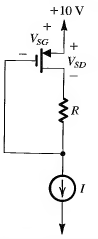Solution:

VSG will be equal to VSD only when the resistance shown is zero or in other words there should not be any resistance.